### 15.4.2.3 Lie brackets

The key to establishing whether a system is nonholonomic is to construct motions that combine the effects of two action variables, which may produce motions in a direction that seems impossible from the system distribution. To motivate the coming ideas, consider the differential-drive model from (15.54). Apply the following piecewise-constant action trajectory over the interval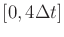: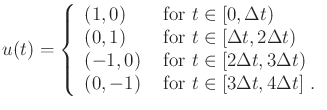(15.71)

The action trajectory is a sequence of four motion primitives: 1) translate forward, 2) rotate forward, 3) translate backward, and 4) rotate backward.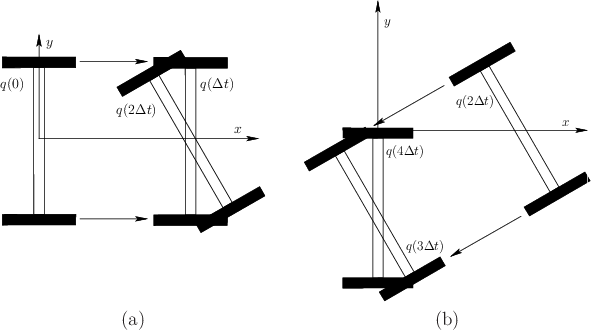The result of all four motion primitives in succession from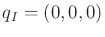is shown in Figure 15.16. It is fun to try this at home with an axle and two wheels (Tinkertoys work well, for example). The result is that the differential drive moves sideways!15.9 From the transition equation (15.54) such motions appear impossible. This is a beautiful property of nonlinear systems. The state may wiggle its way in directions that do not seem possible. A more familiar example is parallel parking a car. It is known that a car cannot directly move sideways; however, some wiggling motions can be performed to move it sideways into a tight parking space. The actions we perform while parking resemble the primitives in (15.71).

Algebraically, the motions of (15.71) appear to be checking for commutativity. Recall from Section 4.2.1 that a group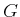is called commutative (or Abelian) if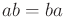for any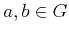. A commutator is a group element of the form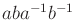. If the group is commutative, then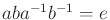(the identity element) for any. If a nonidentity element ofis produced by the commutator, then the group is not commutative. Similarly, if the trajectory arising from (15.71) does not form a loop (by returning to the starting point), then the motion primitives do not commute. Therefore, a sequence of motion primitives in (15.71) will be referred to as the commutator motion. It will turn out that if the commutator motion cannot produce any velocities not allowed by the system distribution, then the system is completely integrable. This means that if we are trapped on a surface, then it is impossible to leave the surface by using commutator motions.

Now generalize the differential drive to any driftless control-affine system that has two action variables: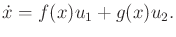(15.72)

Using the notation of (15.53), the vector fields would be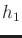and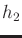; however,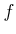and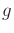are chosen here to allow subscripts to denote the components of the vector field in the coming explanation.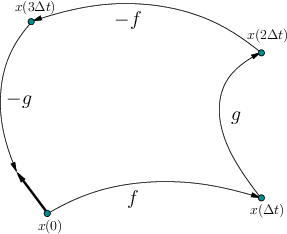Suppose that the commutator motion (15.71) is applied to (15.72) as shown in Figure 15.17. Determining the resulting motion requires some general computations, as opposed to the simple geometric arguments that could be made for the differential drive. If would be convenient to have an expression for the velocity obtained in the limit as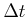approaches zero. This can be obtained by using Taylor series arguments. These are simplified by the fact that the action history is piecewise constant.

The coming derivation will require an expression for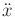under the application of a constant action. For each action, a vector field of the form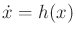is obtained. Upon differentiation, this yields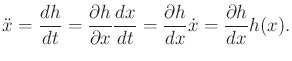(15.73)

This follows from the chain rule because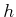is a function of, which itself is a function of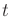. The derivative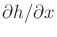is actually an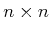Jacobian matrix, which is multiplied by the vector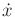. To further clarify (15.73), each component can be expressed as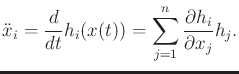(15.74)

Now the state trajectory under the application of (15.71) will be determined using the Taylor series, which was given in (14.17). The state trajectory that results from the first motion primitive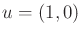can be expressed as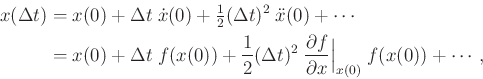(15.75)

which makes use of (15.73) in the second line. The Taylor series expansion for the second primitive is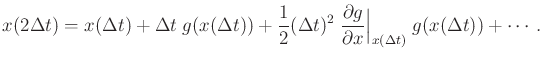(15.76)

An expression for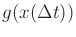can be obtained by using the Taylor series expansion in (15.75) to express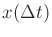. The first terms after substitution and simplification are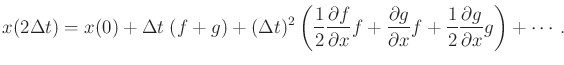(15.77)

To simplify the expression, the evaluation at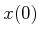has been dropped from every occurrence ofandand their derivatives.

The idea of substituting previous Taylor series expansions as they are needed can be repeated for the remaining two motion primitives. The Taylor series expansion for the result after the third primitive is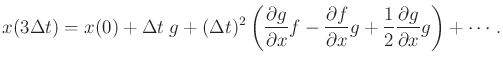(15.78)

Finally, the Taylor series expansion after all four primitives have been applied is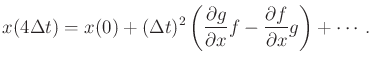(15.79)

Taking the limit yields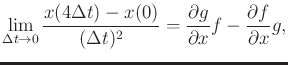(15.80)

which is called the Lie bracket ofandand is denoted by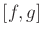. Similar to (15.74), theth component can be expressed as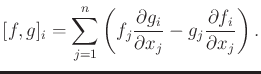(15.81)

The Lie bracket is an important operation in many subjects, and is related to the Poisson and Jacobi brackets that arise in physics and mathematics.

Example 15..9 (Lie Bracket for the Differential Drive)   The Lie bracket should indicate that sideways motions are possible for the differential drive. Consider taking the Lie bracket of the two vector fields used in (15.54). Let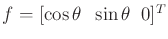and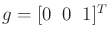. Renameandtoandto allow subscripts to denote the components of a vector field.

By applying (15.81), the Lie bracketis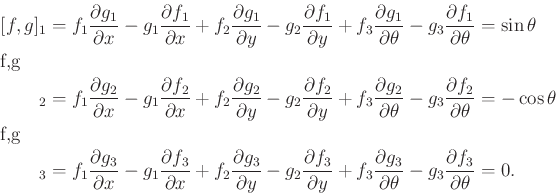(15.82)

The resulting vector field is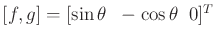, which indicates the sideways motion, as desired. When evaluated at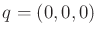, the vector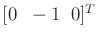is obtained. This means that performing short commutator motions wiggles the differential drive sideways in the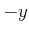direction, which we already knew from Figure 15.16.Example 15..10 (Lie Bracket of Linear Vector Fields)   Suppose that each vector field is a linear function of. TheJacobians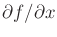and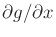are constant.

As a simple example, recall the nonholonomic integrator (13.43). In the linear-algebra form, the system is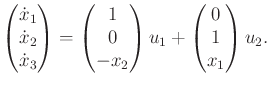(15.83)

Let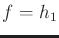and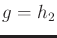. The Jacobian matrices are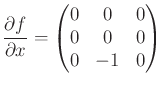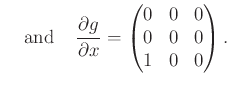(15.84)

Using (15.80),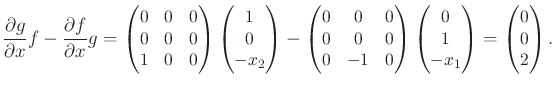(15.85)

This result can be verified using (15.81).Steven M LaValle 2020-08-14